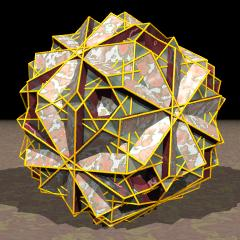Acronym gaquatid TOCID symbol tJE* Name great quasitruncated icosidodecahedron,stellatruncated icosidodecahedron `© ©` Circumradius sqrt[31-12 sqrt(5)]/2 = 1.020684 Vertex figure [10/3,4,6] General of army v3x5f Colonel of regiment (is itself locally convex – no other uniform polyhedral members) Dihedral angles between {4} and {10/3}:   arccos(-sqrt[(5-sqrt(5))/10]) = 121.717474° between {6} and {10/3}:   arccos(sqrt[(5-2 sqrt(5))/15]) = 79.187683° between {4} and {6}:   arccos(sqrt[(3-sqrt(5))/6]) = 69.094843° ExternallinksAs abstract polytope gaquatid is isomorphic to grid, thereby replacing decagrams by decagons.

Incidence matrix according to Dynkin symbol

```x5/3x3x

.   . . | 120 |  1  1  1 |  1  1  1
--------+-----+----------+---------
x   . . |   2 | 60  *  * |  1  1  0
.   x . |   2 |  * 60  * |  1  0  1
.   . x |   2 |  *  * 60 |  0  1  1
--------+-----+----------+---------
x5/3x . |  10 |  5  5  0 | 12  *  *
x   . x |   4 |  2  0  2 |  * 30  *
.   x3x |   6 |  0  3  3 |  *  * 20

snubbed forms: s5/3s3s
```

 © 2004-2019 top of page